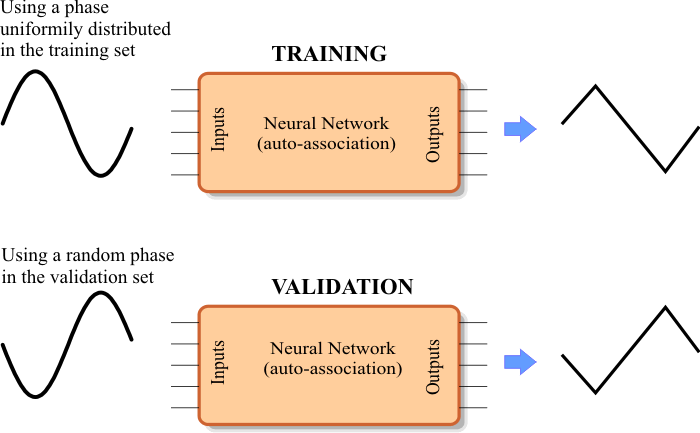# Wave Converter

 Problem 1 Create a New Project called WaveConverter to build an appropriate training set to use an ANN as a wave converter from sine wave to triangular. Use a uniformly distributed phase for each training case.Problem 2 Edit the BuilValidSet.lab file to build the validation set using the training set using random phase for each validation case.

 Problem 3 Edit the Train.lab file to design and train an ANN to convert a sine wave to a triangular wave. Train the ANN using simulated annealing and the conjugate gradient method.

 Problem 4 Edit the CheckTraining.lab file to check the training: (a) Compute the mean squared error for the ANN using the training set. (b) Plot the error for each training case. (c) Save the plot as a vector image (checkTraining.pdf and checkTraining.emf)

 Problem 5 Edit the Validation.lab file to perform the validation of the ANN. (a) Compute the mean squared error for the ANN using the validation set. (b) Plot the error for each validation case. (c) Save the plot as a vector image (validation.pdf and validation.emf)

 Problem 6 Generate a report in Microsoft Word. Write some conclusions in the report focusing on the problems that were faced during the simulation and how these problems were or could be solved.